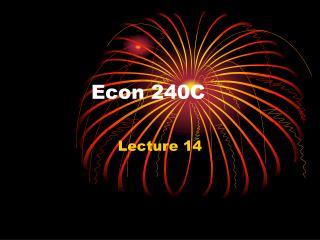DownloadDownload PresentationEcon 240C

# Econ 240C

Télécharger la présentation## Econ 240C

- - - - - - - - - - - - - - - - - - - - - - - - - - - E N D - - - - - - - - - - - - - - - - - - - - - - - - - - -
##### Presentation Transcript

1. Econ 240C Lecture 14

2. ARCH-GARCH Structure?

3. Outline • Part I: Conditional Heteroskedasticity: example • Part II: Detecting ARCH • Part III: Modeling ARCH & GARCH • Part IV: Estimating GARCH

4. Part I. Conditional Heteroskedasticity • An Example

5. Producer Price Index for Finished Goods • April 1947-April 2007 • 1982=100 • Seasonally adjusted rate (SAR)

6. Transformations • PPI is evolutionary • Take logarithms • Then difference • Obtain the fractional changes, i.e. the inflation rate for producer goods

7. Stagflation of the 70’s

8. Modeling dlnppi • Try an arthree

9. Modeling dlnppi • Try an ARMA(1,1)

10. ARMA(1,1) Model of Producer Goods Inflation • Residuals from ARMA(1, 1) model are approximately orthogonal but not normal • Are we done?

11. Part II. Examine Residuals • Trace of residuals • Trace of square of residuals

12. Resq =resid*resid

13. Episodic variance • Not homoskedastic • So call heteroskedastic, conditional on dates or episodes when the variance kicks up • Hence name “conditional heteroskedaticity”

14. Clues • Check trace of residuals squared • Check correlogram of residuals squared • Equation window: View menu, residuals • Check ARCH Lagrange Multiplier Test

15. ARCH Lagrange Multiplier Test

16. Clues • Check trace of residuals squared • can get residuals from Actual, fitted, residuals table • Check correlogram of residuals squared • EVIEWS option along with correlogram of residuals • Heteroskedasticity of residuals • Histogram of residuals • kurtotic residuals are a clue

17. How should we model the conditional heteroskedasticity?

18. Part III: Modeling Conditional Heteroskedasticity • Robert Engle: UCSD • Autoregressive error variance model

19. Modeling the error • Model the error e(t) as the product of two independent parts, wn(t) and h(t) • WN(t) ~N(0,1)

20. Modeling the error • Assume that WN(t) is independent of • So density f{wn(t)*[h(t)]1/2} is the product of two densities, g and k: • f =g[wn(t)]*k{[h(t)]1/2} • And expectations can be written as products of expectations • This is related to writing the Probability of P(A and B) as P(A)*P(B) if events A • And B are independent

21. Modeling the error • We would like the error, e(t) to have the usual properties of mean zero, orthogonality, and unconditional variance constant • E e(t) = E {[h(t)]1/2*WN(t)} = E{[h(t)]1/2}*E[WN(t)] , the product of expectations because of independence • We may not know E{[h(t)]1/2}, but we know E[WN(t)] =0 so Ee(t)=0